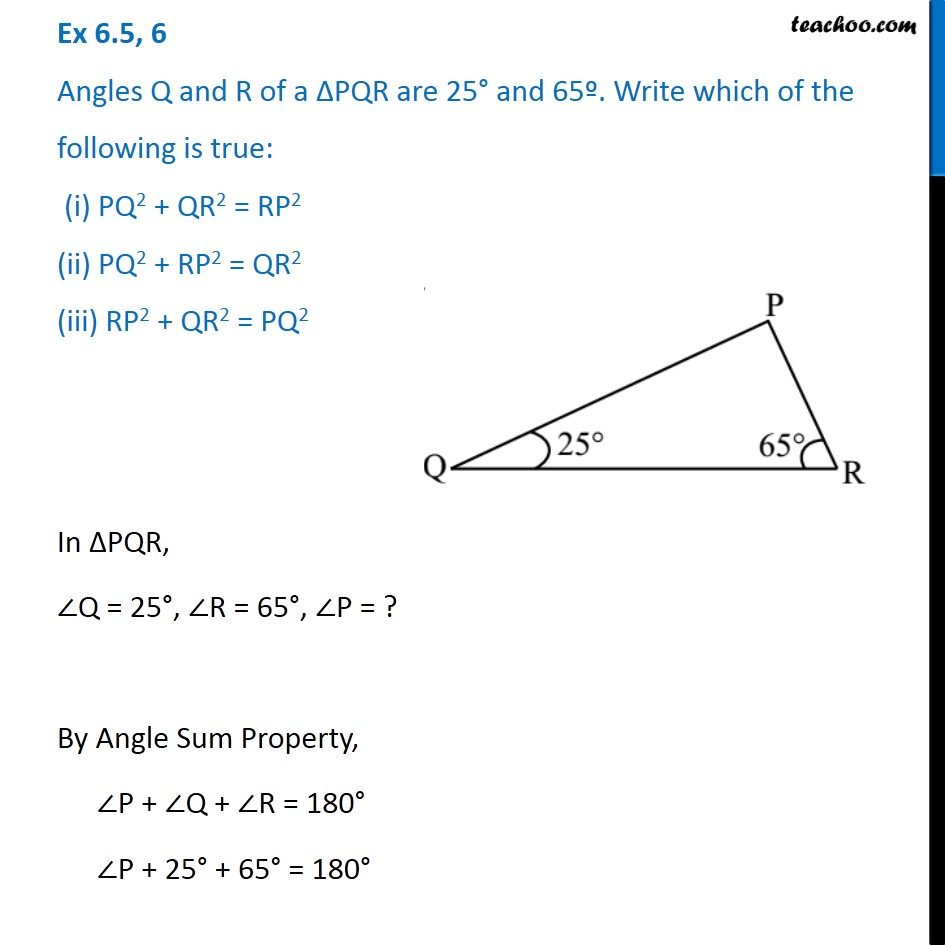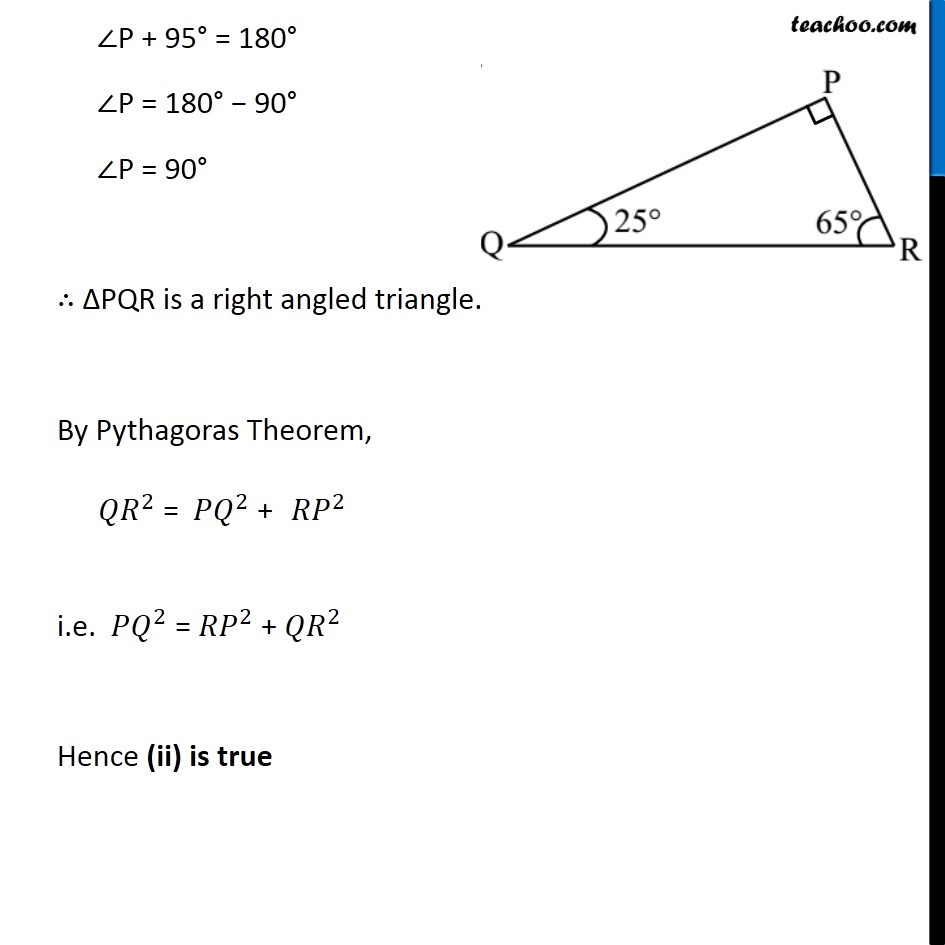1. Chapter 6 Class 7 Triangle and its Properties
2. Concept wise
3. Pythagoras Theorem

Transcript

Ex 6.5, 6 Angles Q and R of a ∆PQR are 25° and 65º. Write which of the following is true: (i) PQ2 + QR2 = RP2 (ii) PQ2 + RP2 = QR2 (iii) RP2 + QR2 = PQ2 In ∆PQR, ∠Q = 25°, ∠R = 65°, ∠P = ? By Angle Sum Property, ∠P + ∠Q + ∠R = 180° ∠P + 25° + 65° = 180° ∠P + 95° = 180° ∠P = 180° − 90° ∠P = 90° ∴ ∆PQR is a right angled triangle. By Pythagoras Theorem, i.e. 〖𝑃𝑄〗^2 = 〖𝑅𝑃〗^2 + 〖𝑄𝑅〗^2 Hence (ii) is true

Pythagoras Theorem